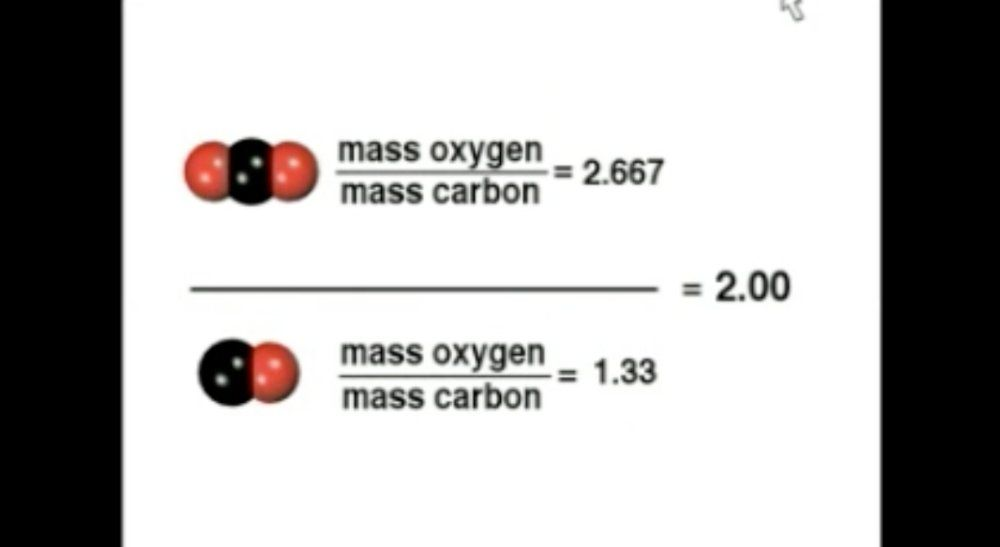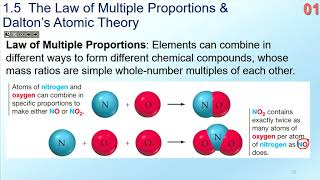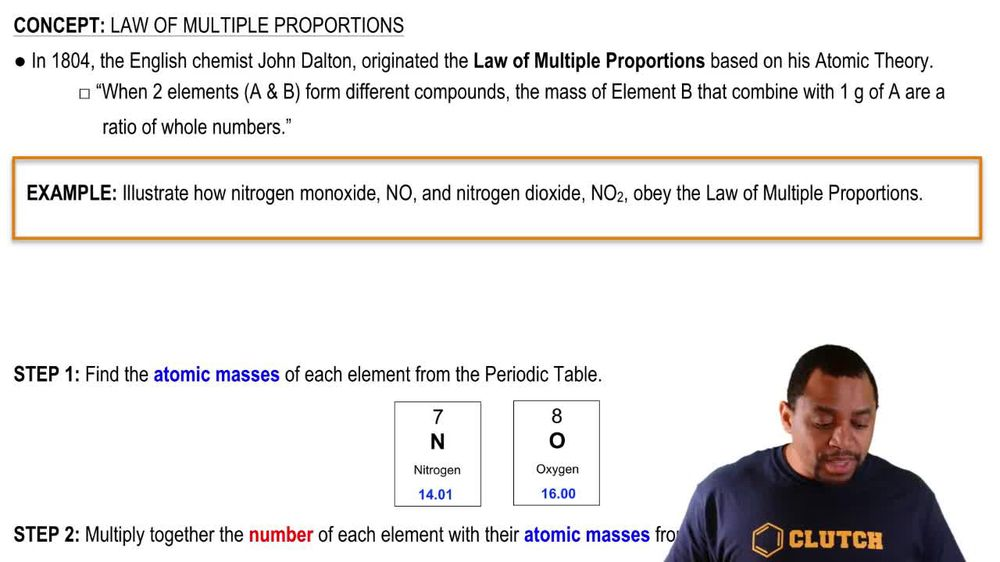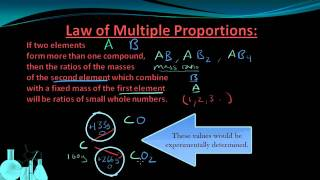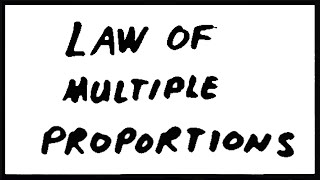Start typing, then use the up and down arrows to select an option from the list.
1. 2. Atoms & Elements2. Law of Multiple Proportions
Problem

# Palladium forms three different compounds with sulfur. The mass of sulfur per gram of palladium in each compound is listed here. Show that these masses are consistent with the law of multiple proportions. Compound Grams S per Gram Pd A 0.603 B 0.301 C 0.151

Relevant Solution2m
Play a video:
Hey everyone. This problem tells us that compound a contains . sulfur program of platinum and compound B contains 0.712 sulfur program of platinum. We need to determine if these amounts follow the law of multiple proportions and explain why. So let's start off by talking about what is the law of multiple proportions. It tells us that when two elements combined with each other, in this case we do have two elements, we have sulfur and we have platinum. So when two elements combine with each other to form two or more compounds. And in this case we have two compounds, we have compound A. And compound B. The ratio is a simple whole number between them. So what we want to do is see what the ratio is between compound A. And compound B. So if we take the ratio compound B over compound A. This ratio should give us a simple whole number and this is what the law of multiple proportions tells us. So we have 0. over 0.356. When we do this calculation we get the # two. And this follows the law of multiple proportions. Because the ratio of sulfur per gram of platinum of the two compounds is a whole number. So to is our race our whole number and it does follow the law of multiple proportions. So that is the end of this problem. I hope this was helpful